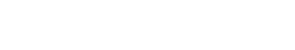# G-and-H Distribution: Simple Definition

The g-and-h distribution (a combination of the g-distribution and h-distribution*) is used in statistical modeling as an alternative for Ordinary Least Squares. Developed by Tukey in 1977, it is seldom used perhaps because it does not have a closed-form solution for a probability density function (PDF). Turley (n.d.) states that as the distribution is so difficult to compute, it has very little practical use. However, it is sometimes used as a model for a severity distribution.

## Calculating the G-and-H Distribution’s Parameters

The g-and-h distribution’s parameters can only be calculated by indirect methods like ones based on order statistics Dutta and Babbel (2005) or method of moments / maximum likelihood estimation Turley, P (n.d.).

The g-and-h distribution can be defined by its moments , although requires some heavy calculus. The scope of this is beyond this article, but details of deriving the first few moments can be found in Cruz et. al’s Fundamental Aspects of Operational Risk and Insurance Analytics: A Handbook (pp. 318-319).

Some formulas are available for very particular random variables. For example, Chaudhuri and Ghosh offer the following formula for the g-and-h distribution for a univariate normal random variable Yg,h, defined by a Z-transformation:Where:

* The g-distribution and h-distrubution can both be derived from the above equation. The g-distribution is derived when h = 0 in the above equation. It corresponds to a scaled LogNormal distribution when g is a constant.
The h-distribution is obtained when g=0 in the equation.

References:
Chaudhuri, A. & Ghosh, S. Retrieved July 8, 2017 from: Quantitative Modeling of Operational Risk in Finance and Banking Using Possibility Theory https://books.google.com/books?id=HMXYCgAAQBAJ&pg=PA29&dq=g-and-h+distribution&hl=en&sa=X&ved=0ahUKEwjJ76v76vnUAhWB6oMKHT7_A2AQ6AEIJDAA#v=snippet&q=g-and-h%20distribution&f=false
Dutta, K.K. and D.F. Babel (2002). Extracting Probabilistic Information fro the Prices of Interested Rate Options: Test of Distributional Assumptions. The Journal of Business 78(3), 841-870.
Tukey, J. W. (1977). Exploratory Data Analysis. Reading, MA: Addison-Wesley
Turley, P. Just a few more moments: the g-and-h distribution. Retrieved July 8, 2017 from: https://www.researchgate.net/publication/251947280_Just_a_few_more_moments_the_g-and-h_distribution

CITE THIS AS:
Stephanie Glen. "G-and-H Distribution: Simple Definition" From StatisticsHowTo.com: Elementary Statistics for the rest of us! https://www.statisticshowto.com/g-and-h-distribution/
---------------------------------------------------------------------------Need help with a homework or test question? With Chegg Study, you can get step-by-step solutions to your questions from an expert in the field. Your first 30 minutes with a Chegg tutor is free!